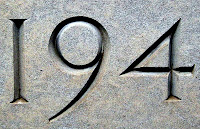## Wednesday, November 25, 2009

### 194

194 = 2 x 97.

194 has a representation as a sum of two squares: 194 = 52 + 132.

194 is the smallest number with five representations as a sum of three squares: 194 = 12 + 72 + 122 = 32 + 42 + 132 = 32 + 82 + 112 = 52 + 52 + 122 = 72 + 82 + 92.

194 is a number n such that n4 + 1 is prime: 1,416,468,497 is prime.194 Prokne is a large, dark, main-belt asteroid discovered in 1879.

Source: